RS Aggarwal Class 10 Solutions Chapter 14 - Height And Distance Ex 14A (14.1)

RS Aggarwal Class 10 Chapter 14 - Height And Distance Ex 14A (14.1) Solutions Free PDF

Q.1: The angle of elevation of an airplane from a point on the ground is $60^{\circ}$. After flying for 15 seconds, the elevation changes to $30^{\circ}$. If the airplane is flying at a height of 1500 meters, find the speed of the airplane.

Sol:

Let O and B the two positions of the jet plane and let A be the point of observation.

Let AX be the horizontal ground.

Draw OC ⊥ AX and BD ⊥ AX.

Then, ∠CAO = 60°, ∠DAB = 30° and OC = BD = 1500√3 m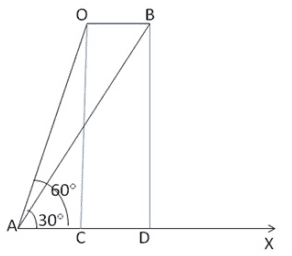From right ∆ OCA, we have

$\frac{AC}{OC}$ = cot 60° = $\frac{1}{√3}$

$\frac{AD}{1500√3}$ = √3 =>AD = (1500√3 x √3) = 4500m

CD = (AD – AC) = (4500 – 1500)m =>3000m

OB = CD = 3000m

Thus, the airplane covers 3000 m in 15 seconds

= ($\frac{3000}{15}$ x $\frac{60 x 60}{1000}$) kmph

Hence the speed of the airplane is = 720kmph.

Q.2: From the top of a building AB, 60m high the angles of depression of the top and bottom of a vertical lamp post CD are observed to be $30^{\circ}\:and\:60^{\circ}$ respectively. Find

(i) The horizontal distance between AB and CD

(ii) The height of the lamp post

(iii) The difference between the heights of the building and the lamp post.

Sol:

Let AB be the building and CD be the light house.

AE is drawn the perpendicular to CD.

Now AB = 60 m

∠ADB = 60°, ∠CAE = 30°

Let BD = x m

AE = BD = x m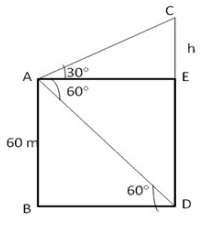In right ∆ACE, let CE = h

$\frac{CE}{AE}$ = tan 30°

$\frac{h}{x}$ = $\frac{1}{√3}$

x = √3h . . . . . . . . . . (1)

In right ∆ABD,

$\frac{AB}{BD}$ = tan 60° => $\frac{60}{x}$ = √3

X = $\frac{60}{√3}$ => $\frac{60√3}{3}$ = 20√3

= 20 x 1.732 = 34.64m . . . . . . . . (2)

From (1) and (2),

20√3 = √3h

h = 20m

Hence,

(i) Difference of heights of light house and building = 20m.

(ii) The distance between light house and building = 34.64m.

Q.3: As observed from the top of a light house, 100m above sea level, the angle of depression of a ship, sailing directly towards it, changes from $30^{\circ}\:and\:60^{\circ}$. Determine the distance traveled by ship during the period of observation. [Use $\sqrt{3}=1.732$]

Sol:

Let AB be the light house and let C and D be the positions of the ship.

Let AD =x, CD = y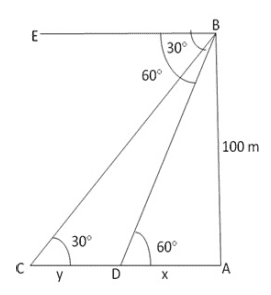In ∆BDA,

$\frac{X}{100}$ = cot 60°

X = $\frac{100}{√3}$m

Similarly in ∆BCA, $\frac{x+y}{100}$ = cot 30°

=> (x+y) = 100√3m

y = (x+y) – x

= ( 100√3 – $\frac{100}{√3}$ )m

= ( $\frac{200}{√3}$ x $\frac{√3}{√3}$ )m = 115.46m

The distance travelled by the ship during the period of observation = 115.46 m

Q. 4: The angle of depression from the top of a tower of a point  A on the ground is $30^{\circ}$. On moving a distance of 20 metres from the point A towards the foot of the tower to a point B, the angle of elevation of the top of the tower from the point B is $60^{\circ}$. Find the height of the tower and its distance from the point A.

Sol:

Let CD be the tower. The angle of elevation from A and B are 60° and 30° respectively.

Let AD = x m and CD = h m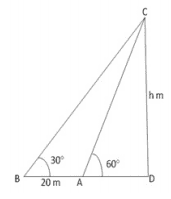In right ∆ ACD,

$\frac{CD}{AD}$ = tan 60°

$\frac{h}{x}$ = √3

h = √3x   ……..(1)

In right angled ∆BCD,

$\frac{CD}{BD}$ = tan 30°

$\frac{h}{20 + x}$ = 1/√3

√3h = 20 + x  ………(2)

Eliminating x from (1) and (2),

√3h = 20 + $\frac{h}{√3}$

or 3h = 20√3 + h

or h = 10√3 => 17.32m

Height of the tower = 17.32 m

Q.5: From the top of a 7-metre-high building, the angle of elevation of the top of the cable tower is $60^{\circ}$ and the angle of depression of its foot is $30^{\circ}$. Determine the height of the tower. [Use $\sqrt{3}=1.732$]

Sol:

Let AB be the building 7 meters high. AE ⊥ CD, where CD is the cable tower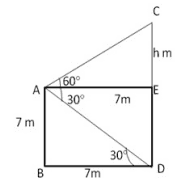In ∆ AED,

∠EAD = 30° = Angle of depression

$\frac{AE}{ED}$ = cot 30°

=> $\frac{x}{7}$ = √3

x = 7√3 m

In  ∆ACE,

∠CAE = 60° => Angle of elevation of C

∠AEC = 90°

$\frac{CE}{AE}$ = tan 60°

$\frac{h}{x}$ = √3

h = √3x

h = √3 x 7√3 => 21m

Height of the tower = CD = CE + ED = (21 + 7) m = 28 m

Q.6: The angles of elevation of a tower from two points at distances of 4m and 9m from the base of the tower and in the same straight line with it are complementary. Show that the height of the tower is 6 meters.

Sol:

Let AB be the tower and let C and D be the two positions of the observer. Then, AC = 9 meters,

Let ∆ ACB = θ

Then, ∠ADB = (90° – θ)

Let AB = h meters

From right ∆ CAB, we have

$\frac{AB}{AC}$ = tan θ

=> $\frac{h}{9}$ = tan θ

h = 9 tan θ

From right ∆ DAB, we have

$\frac{AB}{AD}$ = tan(90° – θ) => $\frac{h}{4}$ = cot θ

=> h = 4 cot θ

from (1) and (2), we get

$h^{2}$ = 36

=> h = 6

Hence, the height of tower is 6 meters.

Q.7: A vertical tower stands on a horizontal plane and is surmounted by a vertical flagstaff of height 10m. At a point on the plane, the angle of elevation of the bottom of the flagstaff is $30^{\circ}$ and that of the top of the flagstaff is $45^{\circ}$. Find the height of the tower. [Use $\sqrt{3}=1.732$]

Sol:

Let P be the point of observation RQ is the building and BR is the flag staff of height h, ∠BPQ = 45°, ∠RPQ = 30°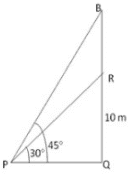$\frac{PQ}{QR}$ = cot 30° = √3

$\frac{PQ}{10}$ = √3

=> PQ = 10√3 m . . . . . . . . . (1)

From the right ∆PBQ, we have

$\frac{PQ}{QB}$ = $\frac{PQ}{10+h}$ = cot 45° = 1

PQ = 10 + h . . . . . . . . (2)

From (1) and (2), we have,

10 + h = 10√3

h = 10√3 – 10 = (10×1.73 – 10)m = (17.3 – 10) = 7.3m

Hence distance of building is and length of the flags staff is 7.3 m.

Q.8: From a point on a bridge across a river, the angles of depression of the banks on opposite sides of the river are $30^{\circ}\:and\:45^{\circ}$ respectively. If the bridge is at a height of 2.5m from the banks, find the width of the river. [Take $\sqrt{3}=1.732$]

Sol:

Let A and B be two points on the bank on opposite sides of the river. Let P be a point on the bridge at a height of 2.5 m

Thus, DP = 2.5 m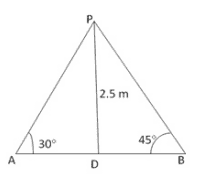Then, ∠BAP = 30°, ∠ABP = 45° and PD = 2.5m

$\frac{DB}{PD}$ = cot 45° =$\frac{DB}{2.5}$ = 1

=> DB = 2.5m

$\frac{AD}{PD}$ = cot 30°

$\frac{AD}{2.5}$ = √3

Height of the river = AB

= (AD + DB) = 2.5(√3 + 1)m = 2.5( 1.732 + 1 )m => 6.83m

Q.9: Two men are on opposite sides of a tower. They measure the angles of elevation of the top of the tower as $30^{\circ}\:and\:45^{\circ}$ respectively. If the height of the tower is 50 metres, find the distance between the two men. [Take $\sqrt{3}=1.732$]

Sol:

Let AB be the tower. Let C and D be the positions of the two men.

Then, ∠ACB = 30°, ∠ADB = 45° and AB = 50 m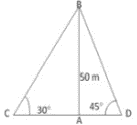$\frac{AC}{AB}$ = cot 30° = √3

=> $\frac{AC}{50}$ = √3

=> AC = 50√3 m

$\frac{AD}{AB}$ = cot 45° = 1

=> $\frac{AD}{50}$ = 1

Distance between the two man = CD = (AC + AD)

= 50(√3 + 1) = 136.6m

Q.10: The horizontal distance between two towers is 60 meters. The angle of depression of the top of the first tower when seen from the top of the second tower is $30^{\circ}$. If the height of the second tower is 90 meters, find the height of the first tower. [Use$\sqrt{3}=1.732$]

Sol:

Let AB and CD be the first and second towers respectively.

Then, CD = 90 m and AC = 60 m.

Let DE be the horizontal line through D.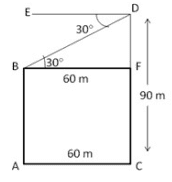Draw BF ⊥ CD,

Then, BF = AC = 60 m

∠FBD = ∠EDB = 30°

Now, $\frac{FD}{BF}$ = tan 30° = $\frac{FD}{60}$ = $\frac{1}{√3}$

=> FD = ( 60 x $\frac{1}{√3}$ )m = 20√3 m

AB = FC = ( CD – FD ) = ( 90 – 20√3 )m = 55.36m

Practise This Question

A population will not exist in Hardy - Weinberg equilibrium if